# class 7 Maths Chapter-10 line and angle

### Exercise-10.1

Question  1:
Draw a line, say AB, take a point C outside it. Through C, draw a line parallel to AB using ruler and compasses only.
Answer: The steps of construction are as follows.
(i)Draw a line AB. Take a point P on it. Take a point C outside this line. Join C to P.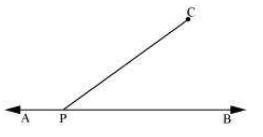(ii)Taking P as centre and with a convenient radius, draw an arc intersecting line AB at point D and PC at point E.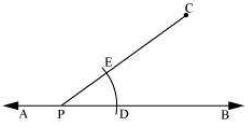(iii) Taking C as centre and with the same radius as before, draw an arc FG intersecting PC at H.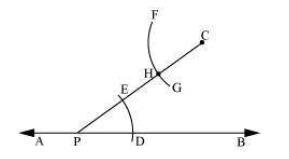(iv) Adjust the compasses up to the length of DE. Without changing the opening of compasses and taking H as the centre, draw an arc to intersect the previously drawn arc FG at point I.

(v) Join the points C and I to draw a line ‘l’.

This is the required line which is parallel to line AB.

Question 2:
Draw a line l. Draw a perpendicular to l at any point on l. On this perpendicular choose a point X, 4 cm away from l. Through X, draw a line m parallel to l.
The steps of construction are as follows.
(i) Draw a line l and take a point P on line l. Then, draw a perpendicular at point P.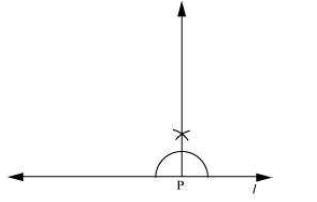(ii) Adjusting the compasses up to the length of 4 cm, draw an arc to intersect this perpendicular at point X. Choose any point Y on line l. Join X to Y.

(iii) Taking Y as centre and with a convenient radius, draw an arc intersecting l at A and XY at B.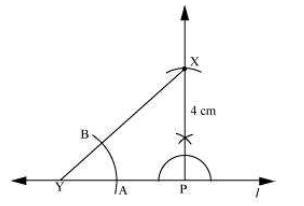(iv) Taking X as centre and with the same radius as before, draw an arc CD cutting XY at E.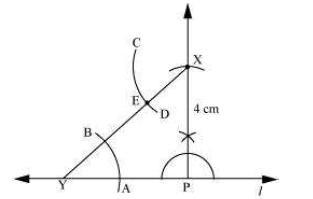(v)Adjust the compasses up to the length of AB. Without changing the opening of compasses and taking E as the centre, draw an arc to intersect the previously drawn arc CD at point F.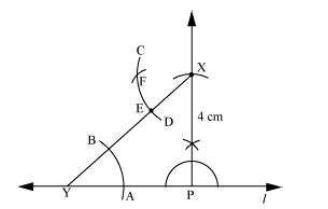(vi) Join the points X and F to draw a line m.

Line m is the required line which is parallel to line l.

Question 3:
Let l be a line and P be a point not on l. Through P, draw a line m parallel to l. Now join P to any point Q on l. Choose any other point R on m. Through R, draw a line parallel to PQ. Let this meet l at S. What shape do the two sets of parallel lines enclose?
The steps of construction are as follows.
(i)Draw a line l and take a point A on it. Take a point P not on l and join A to P.

(ii) Taking A as centre and with a convenient radius, draw an arc cutting l at B and AP at C.

(iii)Taking P as centre and with the same radius as before, draw an arc DE to intersect AP at F.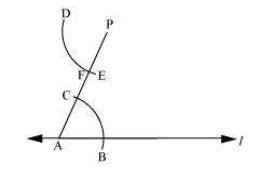(iv) Adjust the compasses up to the length of BC. Without changing the opening of compasses and taking F as the centre, draw an arc to intersect the previously drawn arc DE at point G.

(v)Join P to G to draw a line m. Line m will be parallel to line l.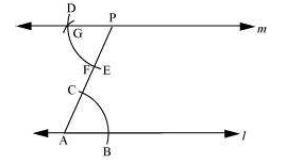(vi)Join P to any point Q on line l. Choose another point R on line m. Similarly, a line can be drawn through point R and parallel to PQ.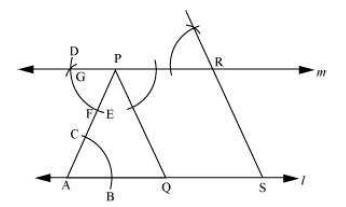Let it meet line l at point S. In quadrilateral PQSR, opposite lines are parallel to each other. PQ || RS and PR || QS Thus, &mnSq1 PQSR is a parallelogram.

### Exercise-10.2

Question 1:
Construct XYZ in which XY = 4.5 cm, YZ = 5 cm and ZX = 6 cm.
The rough figure of this triangle is as follows.

The required triangle is constructed as follows.
(i) Draw a line segment YZ of length 5 cm.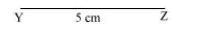(ii) Point X is at a distance of 4.5 cm from point Y. Therefore, taking point Y as centre, draw an arc of 4.5 cm radius.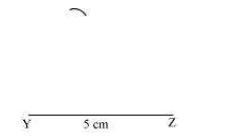(iii) Point X is at a distance of 6 cm from point Z. Therefore, taking point Z as centre, draw an arc of 6 cm radius. Mark the point of intersection of the arcs as X. Join XY and XZ.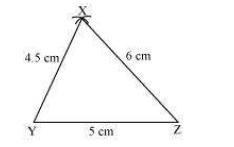XYZ is the required triangle.

Question 2:
Construct an equilateral triangle of side 5.5 cm.
An equilateral triangle of side 5.5 cm has to be constructed. We know that all sides of an equilateral triangle are of equal length. Therefore, a triangle ABC has to be constructed with AB = BC = CA = 5.5 cm. The steps of construction are as follows.
(i) Draw a line segment BC of length 5.5 cm.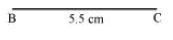(ii) Taking point B as centre, draw an arc of 5.5 cm radius.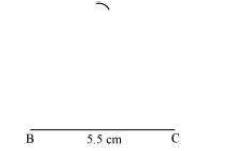(iii) Taking point C as centre, draw an arc of 5.5 cm radius to meet the previous arc at point A.

(iv) Join A to B and C.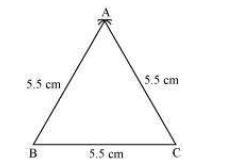ABC is the required equilateral triangle.

Question 3:
Draw PQR with PQ = 4 cm, QR = 3.5 cm and PR = 4 cm. What type of triangle is this?
The steps of construction are as follows.
(i) Draw a line segment QR of length 3.5 cm.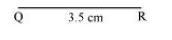(ii) Taking point Q as centre, draw an arc of 4 cm radius.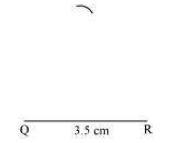(iii) Taking point R as centre, draw an arc of 4 cm radius to intersect the previous arc at point P.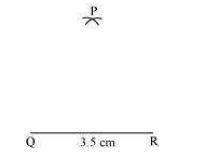(iv) Join P to Q and R.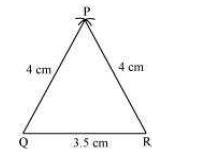PQR is the required triangle. As the two sides of this triangle are of the same length (PQ = PR), therefore, ?PQR is an isosceles triangle.

Question 4:
Construct ABC such that AB = 2.5 cm, BC = 6 cm and AC = 6.5 cm. Measure ∠B.
The steps of construction are as follows.
(i) Draw a line segment BC of length 6 cm.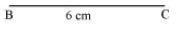(ii) Taking point C as centre, draw an arc of 6.5 cm radius.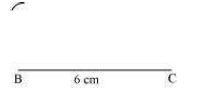(iii) Taking point B as centre, draw an arc of radius 2.5 cm to meet the previous arc at point A.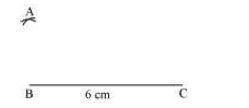(iv) Join A to B and C.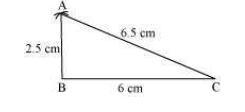ABC is the required triangle. ∠B can be measured with the help of protractor. It comes to 90º.

### Exercise-10.3

Question 1:
Construct DEF such that DE = 5 cm, DF = 3 cm and m∠EDF = 90°.
The rough sketch of the required ?DEF is as follows.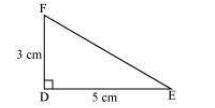The steps of construction are as follows.
(i)Draw a line segment DE of length 5 cm.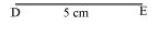(ii) At point D, draw a ray DX making an angle of 90° with DE.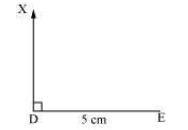(iii) Taking D as centre, draw an arc of 3 cm radius. It will intersect DX at point F.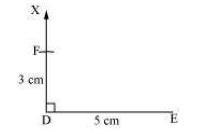(iv) Join F to E. ?DEF is the required triangle.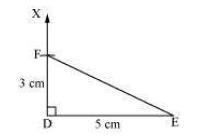Question 2:
Construct an isosceles triangle in which the lengths of each of its equal sides is 6.5 cm and the angle between them is 110°.
An isosceles triangle PQR has to be constructed with PQ = QR = 6.5 cm. A rough sketch of the required triangle can be drawn as follows.

The steps of construction are as follows.
(i) Draw the line segment QR of length 6.5 cm.

(ii) At point Q, draw a ray QX making an angle 110° with QR.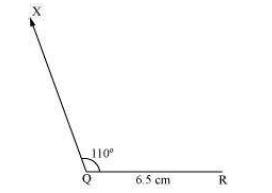(iii) Taking Q as centre, draw an arc of 6.5 cm radius. It intersects QX at point P.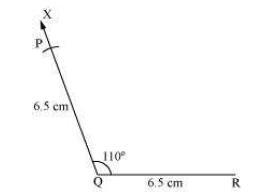(iv) Join P to R to obtain the required triangle PQR.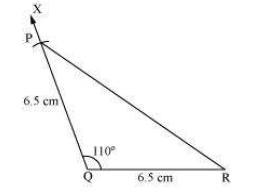Question 3:
Construct ?ABC with BC = 7.5 cm, AC = 5 cm and m∠C = 60°.
A rough sketch of the required triangle is as follows.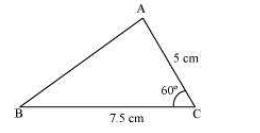The steps of construction are as follows.
(i) Draw a line segment BC of length 7.5 cm.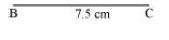(ii) At point C, draw a ray CX making 60º with BC.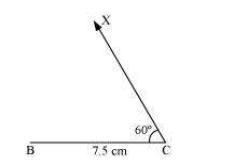(iii) Taking C as centre, draw an arc of 5 cm radius. It intersects CX at point A.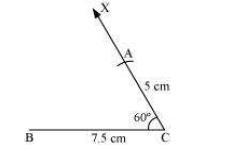(iv) Join A to B to obtain triangle ABC.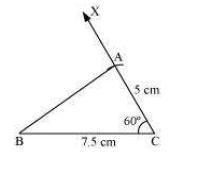### Exercise-10.4

Question 1:
Construct ?ABC, given m∠A = 60°, m∠B = 30° and AB = 5.8 cm.
A rough sketch of the required ?ABC is as follows.

The steps of construction are as follows.
(i)Draw a line segment AB of length 5.8 cm.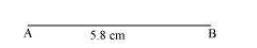(ii)At point A, draw a ray AX making 60º angle with AB.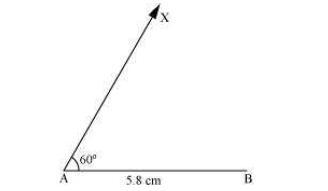(iii) At point B, draw a ray BY, making 30º angle with AB.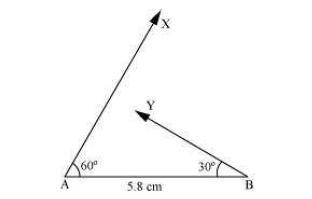(iv) Point C has to lie on both the rays, AX and BY. Therefore, C is the point of intersection of these two rays.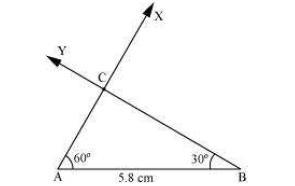This is the required triangle ABC.

Question 2:
Construct ?PQR if PQ = 5 cm, m∠PQR = 105° and m∠QRP = 40°.
(Hint: Recall angle sum property of a triangle).
A rough sketch of the required ?PQR is as follows.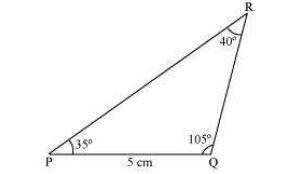In order to construct ?PQR, the measure of ∠RPQ has to be calculated.
According to the angle sum property of triangles,
∠PQR + ∠PRQ + ∠RPQ = 180º
105º + 40º + ∠RPQ = 180º

145º + ∠RPQ = 180º
∠RPQ = 180° − 145° = 35°
The steps of construction are as follows.
(i) Draw a line segment PQ of length 5 cm.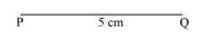(ii) At P, draw a ray PX making an angle of 35º with PQ.

(iii) At point Q, draw a ray QY making an angle of 105º with PQ.

(iv)Point R has to lie on both the rays, PX and QY. Therefore, R is the point of intersection of these two rays.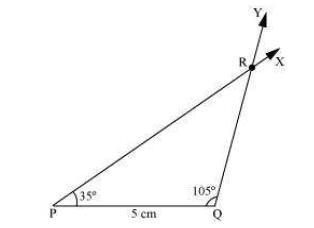This is the required triangle PQR.

Question 3:
Examine whether you can construct DEF such that EF = 7.2 cm, m∠E = 110° and m∠F = 80°. Justify your answer.
Given that,
m∠E = 110° and m∠F = 80°
Therefore,
m∠E + m∠F = 110° + 80° = 190°

However, according to the angle sum property of triangles, we should obtain
m∠E + m∠F + m∠D = 180°
Therefore, the angle sum property is not followed by the given triangle. And thus, we cannot construct ?DEF with the given measurements.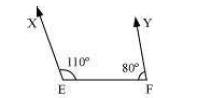Also, it can be observed that point D should lie on both rays, EX and FY, for constructing the required triangle. However, both rays are not intersecting each other. Therefore, the required triangle cannot be formed

### Exercise-10.5

Question 1:
Construct the right angled ?PQR, where m∠Q = 90°, QR = 8 cm and PR = 10 cm.
A rough sketch of ?PQR is as follows.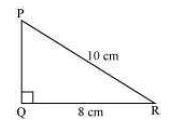The steps of construction are as follows.
(i) Draw a line segment QR of length 8 cm.

(ii) At point Q, draw a ray QX making 90º with QR.

(iii) Taking R as centre, draw an arc of 10 cm radius to intersect ray QX at point P.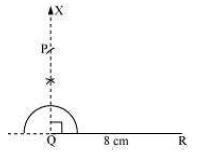(iv) Join P to R. ?PQR is the required right-angled triangle.

Question 2:
Construct a right-angled triangle whose hypotenuse is 6 cm long and one of the legs is 4 cm long.
A right-angled triangle ABC with hypotenuse 6 cm and one of the legs as 4 cm has to be constructed. A rough sketch of ?ABC is as follows.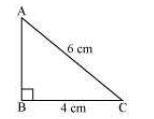The steps of construction are as follows.
(i) Draw a line segment BC of length 4 cm.

(ii) At point B, draw a ray BX making an angle of 90º with BC.

(iii) Taking C as centre, draw an arc of 6 cm radius to intersect ray BX at point A.

(iv) Join A to C to obtain the required ?ABC.

Question 3:
Construct an isosceles right-angled triangle ABC, where, m∠ACB = 90° and AC = 6 cm.
In an isosceles triangle, the lengths of any two sides are equal.
Let in ?ABC, AC = BC = 6 cm. A rough sketch of this ?ABC is as follows.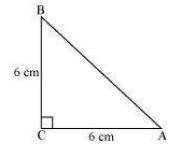The steps of construction are as follows.
(i) Draw a line segment AC of length 6 cm.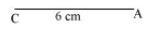(ii) At point C, draw a ray CX making an angle of 90º with AC.

(iii) Taking point C as centre, draw an arc of 6 cm radius to intersect CX at point B.

(iv) Join A to B to obtain the required ?ABC.

Get 30% off your first purchase!

X
error: Content is protected !!
Scroll to Top